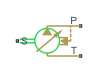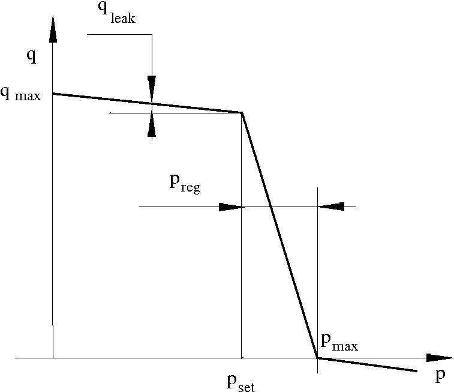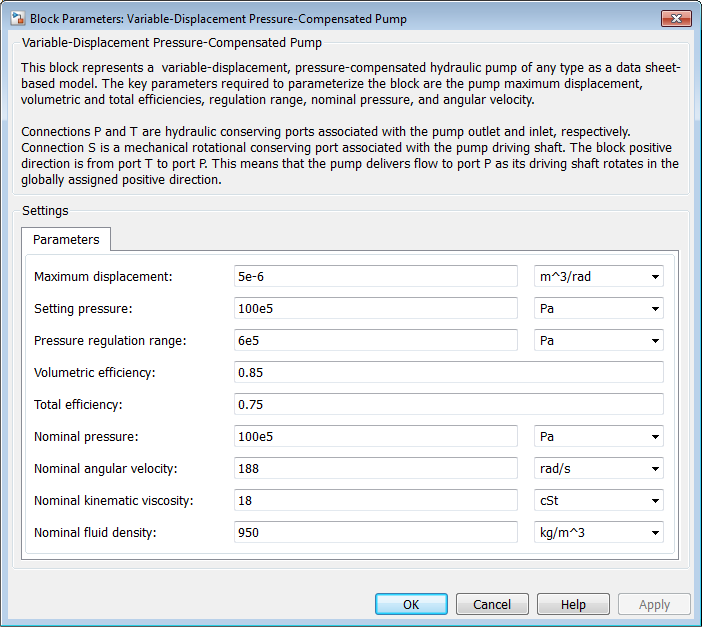# Variable-Displacement Pressure-Compensated Pump

(To be removed) Hydraulic pump maintaining preset pressure at outlet by regulating its flow delivery

The Hydraulics (Isothermal) library will be removed in a future release. Use the Isothermal Liquid library instead. (since R2020a)

## Library

Pumps and Motors

•## Description

The Variable-Displacement Pressure-Compensated Pump block represents a positive, variable-displacement, pressure-compensated pump of any type as a data-sheet-based model. The key parameters required to parameterize the block are the pump maximum displacement, regulation range, volumetric and total efficiencies, nominal pressure, and angular velocity. All these parameters are generally provided in the data sheets or catalogs.

The following figure shows the delivery-pressure characteristic of the pump.The pump tries to maintain preset pressure at its outlet by adjusting its delivery flow in accordance with the system requirements. If pressure differential across the pump is less than the setting pressure, the pump outputs its maximum delivery corrected for internal leakage. After the pressure setting has been reached, the output flow is regulated to maintain preset pressure by changing the pump's displacement. The displacement can be changed from its maximum value down to zero, depending upon system flow requirements. The pressure range between the preset pressure and the maximum pressure, at which the displacement is zero, is referred to as regulation range. The smaller the range, the higher the accuracy at which preset pressure is maintained. The range size also affects the pump stability, and decreasing the range generally causes stability to decrease.

The variable-displacement, pressure-compensated pump is represented with the following equations:

`$q=D\cdot \omega -{k}_{leak}\cdot p$`

`$T=D\cdot p/{\eta }_{mech}$`

`${p}_{\mathrm{max}}={p}_{set}+{p}_{reg}$`

`$K={D}_{\mathrm{max}}/\left({p}_{\mathrm{max}}-{p}_{set}\right)$`

`${k}_{leak}=\frac{{k}_{HP}}{\nu \cdot \rho }$`

`${k}_{HP}=\frac{{D}_{\mathrm{max}}\cdot {\omega }_{nom}\left(1-{\eta }_{V}\right)\cdot {\nu }_{nom}\cdot {\rho }_{nom}}{{p}_{nom}}$`

`$p={p}_{P}-{p}_{T}$`

where

 `q` Pump delivery `p` Pressure differential across the pump `pP,pT` Gauge pressures at the block terminals `D` Pump instantaneous displacement `Dmax` Pump maximum displacement `pset` Pump setting pressure `pmax` Maximum pressure, at which the pump displacement is zero `T` Torque at the pump driving shaft ω Pump angular velocity `kleak` Leakage coefficient `kHP` Hagen-Poiseuille coefficient ηV Pump volumetric efficiency ηmech Pump mechanical efficiency ν Fluid kinematic viscosity ρ Fluid density ρnom Nominal fluid density `pnom` Pump nominal pressure ωnom Pump nominal angular velocity νnom Nominal fluid kinematic viscosity

The leakage flow is determined based on the assumption that it is linearly proportional to the pressure differential across the pump and can be computed by using the Hagen-Poiseuille formula

`$p=\frac{128\mu l}{\pi {d}^{4}}{q}_{leak}=\frac{\mu }{{k}_{HP}}{q}_{leak}$`

where

 `qleak` Leakage flow `d, l` Geometric parameters of the leakage path μ Fluid dynamic viscosity, μ = ν.ρ

The leakage flow at `p` = `pnom` and ν = νnom can be determined from the catalog data

`${q}_{leak}={D}_{\mathrm{max}}\cdot {\omega }_{nom}\left(1-{\eta }_{V}\right)$`

which provides the formula to determine the Hagen-Poiseuille coefficient

`${k}_{HP}=\frac{{D}_{\mathrm{max}}\cdot {\omega }_{nom}\left(1-{\eta }_{V}\right)\cdot {\nu }_{nom}\cdot {\rho }_{nom}}{{p}_{nom}}$`

The pump mechanical efficiency is not usually available in data sheets, therefore it is determined from the total and volumetric efficiencies by assuming that the hydraulic efficiency is negligibly small

`${\eta }_{mech}={\eta }_{total}/{\eta }_{V}$`

The block positive direction is from port T to port P. This means that the pump transfers fluid from T to P provided that the shaft S rotates in the positive direction. The pressure differential across the pump is determined as $p={p}_{P}-{p}_{T}$.

## Basic Assumptions and Limitations

• Fluid compressibility is neglected.

• No loading on the pump shaft, such as inertia, friction, spring, and so on, is considered.

• Leakage inside the pump is assumed to be linearly proportional to its pressure differential.

## Dialog Box and ParametersMaximum displacement

Pump displacement. The default value is `5e-6` m^3/rad.

Setting pressure

Pump pressure setting. The default value is `1e7` Pa.

Pressure regulation range

Pressure range required to change the pump displacement from its maximum to zero. The default value is `6e5` Pa.

Volumetric efficiency

Pump volumetric efficiency specified at nominal pressure, angular velocity, and fluid viscosity. The default value is `0.85`.

Total efficiency

Pump total efficiency, which is determined as a ratio between the hydraulic power at the pump outlet and mechanical power at the driving shaft at nominal pressure, angular velocity, and fluid viscosity. The default value is `0.75`.

Nominal pressure

Pressure differential across the pump, at which both the volumetric and total efficiencies are specified. The default value is `1e7` Pa.

Nominal angular velocity

Angular velocity of the driving shaft, at which both the volumetric and total efficiencies are specified. The default value is `188` rad/s.

Nominal kinematic viscosity

Working fluid kinematic viscosity, at which both the volumetric and total efficiencies are specified. The default value is `18` cSt.

Nominal fluid density

Working fluid density, at which both the volumetric and total efficiencies are specified. The default value is `900` kg/m^3.

## Global Parameters

Parameter determined by the type of working fluid:

• Fluid kinematic viscosity

Use the Hydraulic Fluid block or the Custom Hydraulic Fluid block to specify the fluid properties.

## Ports

The block has the following ports:

`T`

Hydraulic conserving port associated with the pump suction, or inlet.

`P`

Hydraulic conserving port associated with the pump outlet.

`S`

Mechanical rotational conserving port associated with the pump driving shaft.

## Examples

The Closed-Loop Actuator with Variable-Displacement Pressure-Compensated Pump example illustrates the use of the Variable-Displacement Pressure-Compensated Pump block in hydraulic systems.

## Version History

Introduced in R2006a

expand all